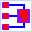#### File Exchange > Data Analysis >    Constrained Multiple RegressionAuthor:
OriginLab Technical Support
1/22/2016
Last Update:
5/8/2017
168
Total Ratings:
1
File Size:
1197 KB
Average Rating:File Name:
Constraine...on.opx
File Version:
1.10
Minimum Versions:
Free
Summary:

Perform constrained multiple regression and solve constrained linear least-squares problems (PRO Only).

Description:

Purpose
This tool can be use to perform constraints multiple linear regression. The following features are supported:

1. Set bounds for each parameter, one by one, or set same bounds for all, including lower bound and upper bound.
2. Set linear constraints. For each linear constraint, you can specify the linear expression, lower bound and upper bound.
NOTE: To force a particular parameter to be positive, you need to set the lower bound to be a very small positive number such as 1E-10.
3. Solve constrained linear least-squares problems with the specified bounds and linear constraints.
4. Output fit parameters and fit statistics.
5. Output fit summary table, ANOVA result, covariance matrix, correlation matrix and fitted values.
6. Output plots including:
• partial leverage plots
• residual vs. independent plot
• histogram of the residual plot
• residual vs predicted values plot
• residual vs. the order of the data plot
• residual lag plot
• composite plot

Installation

2. Drag-and-drop the file onto the Origin workspace. An app icon named Constrained Multiple Regression will appear in the Apps gallery window.

Operation

1. Activate a worksheet with multiple independent variables (X columns) and a dependent variable (Y column).
2. Highlight these columns and click the App icon, or specify the datasets after the dialog box is open.
3. Set bounds for each parameter, together with the linear constraints.
4. Specify output quantities, including parameters, statistics, plots, etc.

 08/06/2021 Ramdhyannishad I want to use